# 漫画：什么是快速排序算法？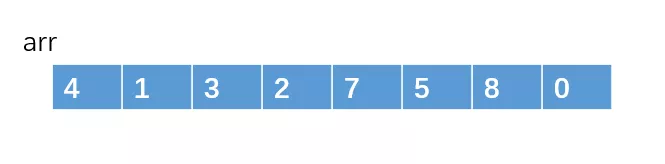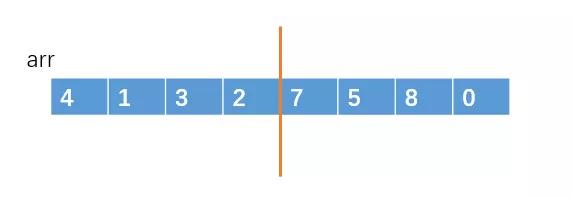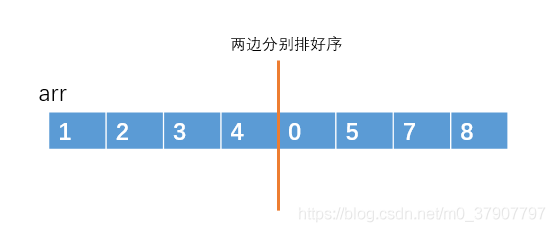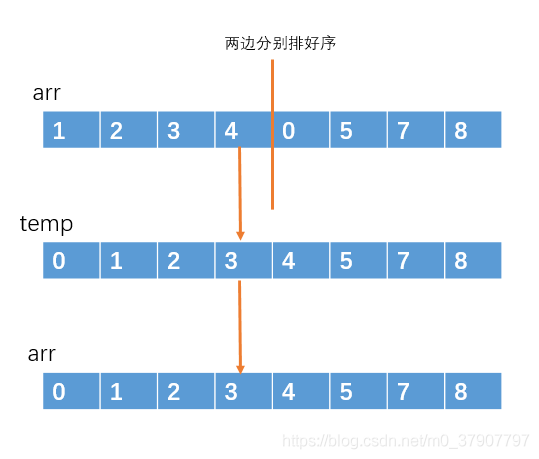#### 快速排序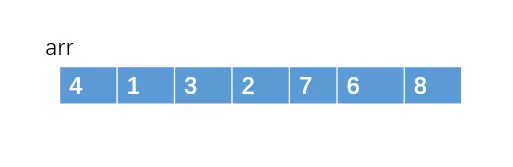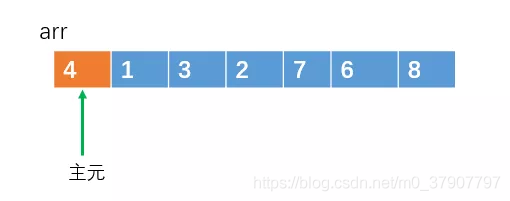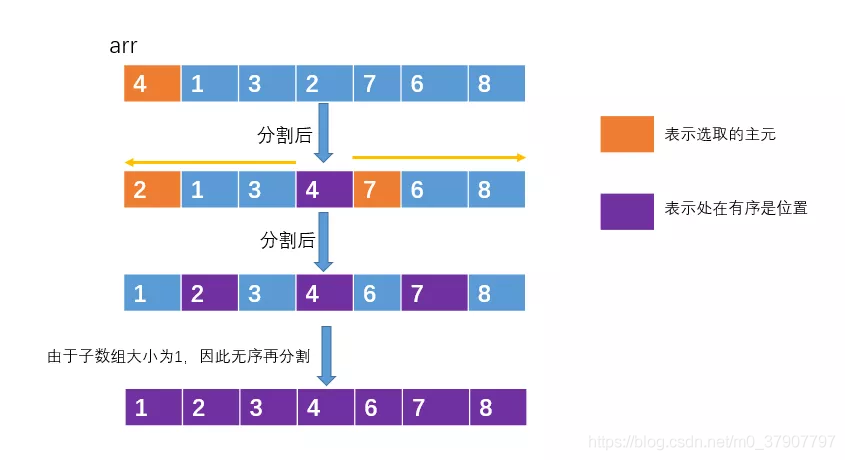#### 分割操作：单向调整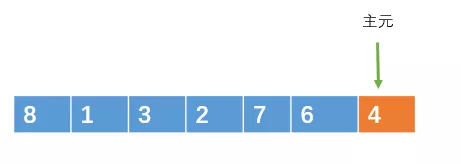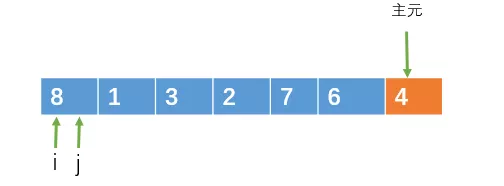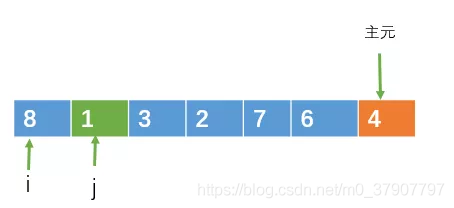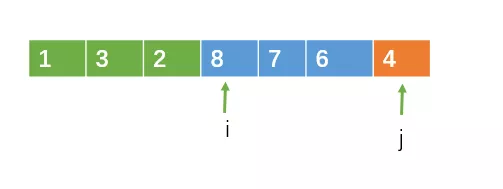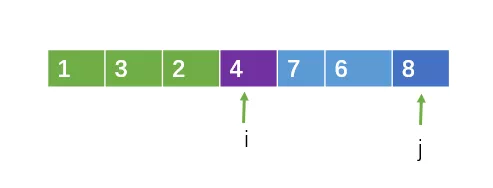//分割操作：方法一，单向调整
int partion(int a[], int left, int right)
{
int temp,pivot;//pivot存放主元
int i,j;
i = left;
pivot = a[right];
for(j = left;j < right;j++)
{
if(a[j] < pivot)
{  //交换值
temp = a[i];
a[i] = a[j];
a[j] = temp;
i++;
}
}
a[right] = a[i];
a[i] = pivot;
return i;//把主元的下标返回
}
//快速排序
void QuickSort(int a[], int left, int right)
{
int center;
int i,j;
int temp;
if(left < right)
{
center = partion(a,left,right);
QuickSort(a,left,center-1);//左半部分
QuickSort(a,center+1,right);//右半部分
}
}


#### 分割操作：双向调整8被重复交换了很多次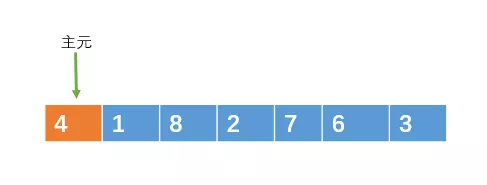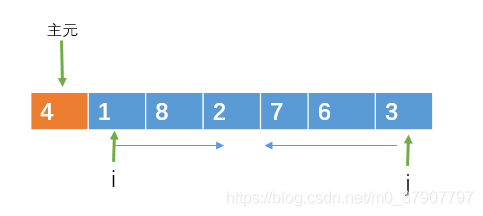i 向右遍历的过程中，如果遇到大于或等于主元的元素时，则停止移动，j向左遍历的过程中，如果遇到小于或等于主元的元素则停止移动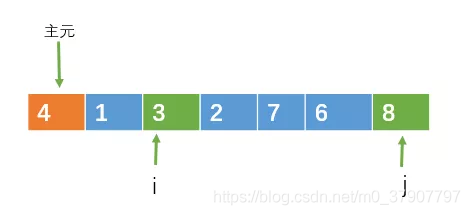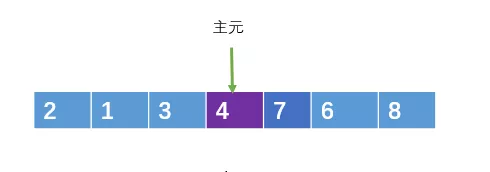方法二：双向扫描
int partition2( int[] arr, int left, int right)
{
int pivot = arr[left];
int i = left + 1;
int j = right;
while(true)
{
//向右遍历扫描
while(i <= j && arr[i] <= pivot) i++;
//向左遍历扫描
while(i <= j && arr[j] => pivot) j--;
if(i >= j)
break;
//交换
int temp = arr[i];
arr[i] = arr[j];
arr[j] = temp;
}
//把arr[j]和主元交换
arr[left] = arr[j];
arr[j] = povit;
return j;
}


#### 时间复杂度#### 稳定性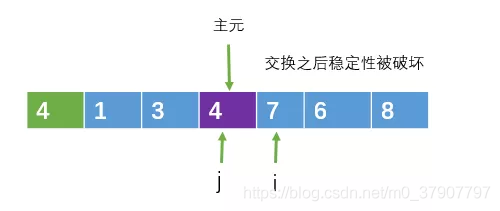//随机选取主元
int random_partition(int[] arr, int left, int right)
{
i = random(left, right);//随机选取一个位置
//在把这个位置的元素与ar[left]交换
swap(arr[i], arr[left]);

return partition(arr, left, right);
}


### 评论(3)

• mpweixin用户 普通 2023年4月27日 上午11:47

我不理解 第二种情况如果主元和i交换了之后，也就是4和7交换，那么交换后不就是 7 1 3 2 4 6 8 那么i前面的元素不满足小于等于i位置上的元素啊

• mpweixin用户 普通 @ mpweixin用户 2023年6月8日 上午11:46

是的赞同，这里细节有点问题，我觉得代码里其实正确的，但是上面的图有点错误，就是ij时，其实已经停止了，这时候i和j相等两个都可以，而不是图中画的继续向后移动

• gdb 普通 2023年3月19日 下午8:48

partion应该是portion吧